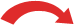# Convert Decimals to Fractions

### To convert a Decimal to a Fraction follow these steps:

• Step 1: Write down the decimal divided by 1, like this:   decimal 1
• Step 2: Multiply both top and bottom by 10 for every number after the decimal point. (For example, if there are two numbers after the decimal point, then use 100, if there are three then use 1000, etc.)
• Step 3: Simplify (or reduce) the fraction

### Example: Convert 0.75 to a fraction

Step 1: Write down 0.75 divided by 1:

0.75 1

Step 2: Multiply both top and bottom by 100 (because there are 2 digits after the decimal point so that is 10×10=100):

 × 1000.75 1 = 75 100× 100

(Do you see how it turns the top number
into a whole number?)

Step 3: Simplify the fraction (this took me two steps):

 ÷5 ÷ 575 100 = 15 20 = 3 4÷5 ÷ 5

Note: 75/100 is called a decimal fraction and 3/4 is called a common fraction !

### Example: Convert 0.625 to a fraction

Step 1: write down:

0.625 1

Step 2: multiply both top and bottom by 1,000 (3 digits after the decimal point, so 10×10×10=1,000)

625 1000

Step 3: Simplify the fraction (it took me two steps here):

 ÷ 25 ÷ 5625 1000 = 25 40 = 5 8÷ 25 ÷ 5

When there is a whole number part, put the whole number aside and bring it back at the end:

### Example: Convert 2.35 to a fraction

Put the 2 aside and just work on 0.35

Step 1: write down:

0.35 1

Step 2: multiply both top and bottom by 100 (2 digits after the decimal point so that is 10×10=100):

35 100

Step 3: Simplify the fraction:

 ÷ 535 100 = 7 20÷ 5

Bring back the 2 (to make a mixed fraction):

### Example: Convert 0.333 to a fraction

Step 1: Write down:

0.333 1

Step 2: Multiply both top and bottom by 1,000 (3 digits after the decimal point so that is 10×10×10=1,000)

333 1000

Step 3: Simplify Fraction:

Can't get any simpler!

## But a Special Note:

If you really meant 0.333... (in other words 3s repeating forever which is called 3 recurring) then we need to follow a special argument. In that case we write down:

0.333... 1

Then multiply both top and bottom by 3:

 × 30.333... 1 = 0.999... 3× 3

And 0.999... = 1 (Does it? - see the 9 Recurring discussion for more if you are interested), so: